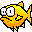# Queues## Mini Lab 1

### A Queue interface, and an implementation

Java does not (yet?) provide a standard Queue interface, but consider the following one.

```/** The Queue interface specifies the methods for a queue data structure.
*    - The enqueue method adds an element to the "back" of the data structure.
*    - The dequeue method acts as follows:
*        - If the queue is empty, an exception is thrown.
*        - If the queue contains one element, dequeue returns that element,
*          leaving the queue empty.
*        - If the queue contains multiple elements, dequeue returns the
*          element at the "front" of the queue, the one that has been there longest.
**/
public interface Queue
{
/** Returns true if this queue is empty; false otherwise. */
boolean isEmpty();

/** Adds a specified object to the "back" of this queue.
*    @param x the object to add to the queue
*/
void enqueue(Object x);

/** Removes the element at the "front" of this queue.
*    @returns the returned element
*    @throws an exception if the queue is empty
**/
Object dequeue();

/** Returns the element at the "front" of this queue, without
*  modifying the queue.
*    @throws an exception if the queue is empty
**/
Object peekFront();

}```

Read through the `Queue` interface and become familiar with the methods and their specifications.

Now consider implementing a class that implements the `Queue` interface with O(1)  performance for all four methods.

#### Analysis Question:

• Consider the following data structures.  Which ones could be used as the internal data representation for a `Queue` implementation with the perfomance characteristics described above?
• a Java array?
• an `ArrayList` object?
• a `LinkedList` object?

Which do you think would be easiest to implement?

#### Exercise:

• Create a class, `LLQueue`, that implements the `Queue` interface using a linked list internally.  Remember that the `LLQueue` class extends `Object` as well as implementing `Queue`, so it inherits methods such as `toString`, `equals`, and `hashCode`, unless it redefines them.  Provide an implementation of the `toString` method that returns a string showing the current state of the queue.

## Mini Lab 2

### Testing and Using `Queue` and `LLQueue`

Client code should be able to use a queue according to the interface, without caring how it is implemented internally.  In fact, well-written client code depends only on the interface, except when constructing the queue.  When an object is constructed, of course, it is necessary to specify the object's class.

For example, consider a variable, called `colorQueue`,  representing a queue containing color information.  The established way of using an interface implementation is to use the interface name for the variable declaration, and to use the actual class name only when constructing the object.   For example, in the statement

```    Queue colorQueue = new LLQueue();
```
`colorQueue` is declared to be a `Queue` (interface), but constructed as an instance of `LLQueue` (implementation). The statement could be written:
```    LLQueue colorQueue = new LLQueue();
```
but this is less flexible.  The first version says, "Create a `Queue` object called `colorQueue`, and implement it using the `LLQueue` class."  The second version says, "Create an `LLQueue` object."   The first version is the accepted pattern unless the client code needs to use methods specific to the actual implementation.

#### Exercise

• Create a test driver for the `LLQueue` class and add three different strings to the queue. You can give the strings whatever values you like: this is for practice.

• (Analysis Question) What happens when you ask for the queue's size? Should there be a `size` method for a queue? If so, where would you put it? If you would like to see the entire contents of the queue, you can use the `toString` method.

• Remove an element from the queue.  What should the queue look like now?

• Remove the rest of the elements in the queue. You could just call the `dequeue` twice more, but what if you have been adding and subtracting elements and don't know how many elements are in the queue?  The elementary pattern for removing all the elements from a queue is similar to the pattern for traversing a linear data structure using an iterator.
```    while ( ! isEmpty() )
{
SpecificType element = (SpecificType) theQueue.dequeue();
// use element in some way
}```
Look over the pattern and make sure that it makes sense to you and that you could use it in code of your own.  Then use it to remove the elements from your queue.

• Add several new items to the queue. Verify that the queue contains what you think it does.